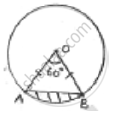Share

# A Chord Ab of Circle, of Radius 14cm Makes an Angle of 60° at the Centre. Find the Area of Minor Segment of Circle. - CBSE Class 10 - Mathematics

ConceptAreas of Sector and Segment of a Circle

#### Question

A chord AB of circle, of radius 14cm makes an angle of 60° at the centre. Find the area of minor segment of circle.

#### SolutionGiven radius (r) = 14cm = OA = OB

𝜃 = angle at centre = 60°

In ΔAOB, ∠A = ∠B     [angles opposite to equal sides OA and OB] = x

By angle sum property ∠A + ∠B + ∠O = 180°

x + x + 60° = 180° ⇒ 2x = 120° ⇒ x = 60°

All angles are 60°, OAB is equilateral OA = OB = AB

Area of segment = area of sector – area Δle OAB

=theta/360^@× pir^2 − sqrt(3)/4× (−AB)^2

=60/360×22/7× 14 × 14 − sqrt(3)/4× 14 × 14

=308/3− 49sqrt(3)

= (308−147sqrt(3))/3cm^2

Is there an error in this question or solution?

#### APPEARS IN

Solution A Chord Ab of Circle, of Radius 14cm Makes an Angle of 60° at the Centre. Find the Area of Minor Segment of Circle. Concept: Areas of Sector and Segment of a Circle.
S